# The algorithms involved in finding a solution with numerical methods

what are all the algorithms involved in finding a solution with numerical methods ?

an example

say we were to solve the initial value problem:

Y′ = 2x

y(0) = 0

it's so simple, you could find a formulaic solution in your head, namely y = x2. On the other hand, say we were to use a numerical technique. (yes, i know we don't know how to do this yet, but go with me on this for a second!) the resulting numerical solution would simply be a table of values. To get a better feel for the nature of these two types of solution, let's compare them side by side, along with the graphs we would get based on what we know about each one:

Notice that the graph derived from the formulaic solution is smoothly continuous, consisting of an infinite number of points on the interval shown. On the other hand, the graph based on the numerical solution consists of just a bare eight points, since the numerical method used apparently only found the value of the solution for x-increments of size 0.2.

Using numerical solutions

so what good is the numerical solution if it leaves out so much of the real answer? Well, we can respond to that question in several ways:

The numerical solution still looks like it is capturing the general trend of the "real" solution, as we can see when we look at the side-by-side graphs. This means that if we are seeking a qualitative view of the solution, we can still get it from the numerical solution, to some extent.

The numerical solution could even be "improved" by playing "join-the-dots" with the set of points it produces. In fact this is exactly what some solver packages, such as mathematica, do do with these solutions. (mathematica produces a join-the-dots function that it calls interpolatingfunction.)

when actually using the solutions to differential equations, we often aren't so much concerned about the nature of the solution at all possible points. Think about it! Even when we are able to get formulaic solutions, a typical use we make of the formula is to substitute values of the independent variable into the formula in order to find the values of the solution at specific points. Did you hear that? Let me say it again: To find the values of the solution at specific points. This is exactly what we can still do with a numerical solution

numerical methods and errors
interpolation
numerical differentiation
numerical integration
solution of algebraic and transcendental equations
numerical solution of a system of linear equations
numerical solution of ordinary differential equations
curve fitting
numerical solution of problems associated with partial differential equations

fixed point iteration method
bisection and regula false methods
newton raphson method etc.
Finite differences operators
numerical interpolation
newton’s and lagrangian formulae
part i
newton’s and lagrangian formulae
part ii
interpolation by iteration
numerical differentiaton
numerical integration
solution of system of linear
equations
solution by iterations
eigen values
taylor series method
picard’s iteration method
euler methods
runge – kutta methods
predictor and corrector methods

Last edited by a moderator:

BvU
Homework Helper
what are all the algorithms involved in finding a solution with numerical methods ?
??
Well, you've listed quite a bunch of them already. Do you expect someone in PF to complete your list? Or is it in fact domething else you want to know ?

BvU ,

i am so confused right now...

A numerical iteration method or simply iteration method is a mathematical
procedure that generates a sequence of improving approximate solutions for a class of
problems. A specific way of implementation of an iteration method, including the
termination criteria, is called an algorithm of the iteration method. In the problems of
finding the solution of an equation an iteration method uses an initial guess to generate
successive approximations to the solution.

what is this initial guess ??
the initial guess of what ??

BvU
Homework Helper
A numerical integration has to start with some value (the initial value, e.g. y(0) = 0) and can then proceed. Good for initial value problems.

When you solve a boundary problem (e.g. y(10) = 100 as a given) using numerical integration, you still have to start somewhere, so you 'guess' the initial value and see where you end up with the integration. (Look up 'shooting method')

these numerical type questions are a bit hard usually ...
i really thought first we had to try to solve the equation in an ,analytic , symbolic or formulaic way ...before trying the numerical way ...

so it means, when i encounter a question like this .. i have to take an initial value ...

an example

say we were to solve the initial value problem:

Y′ = 2x

y(0) = 0

it's so simple, you could find a formulaic solution in your head, namely y = x2. On the other hand, say we were to use a numerical technique. (yes, i know we don't know how to do this yet, but go with me on this for a second!) the resulting numerical solution would simply be a table of values. To get a better feel for the nature of these two types of solution, let's compare them side by side, along with the graphs we would get based on what we know about each one:

Notice that the graph derived from the formulaic solution is smoothly continuous, consisting of an infinite number of points on the interval shown. On the other hand, the graph based on the numerical solution consists of just a bare eight points, since the numerical method used apparently only found the value of the solution for x-increments of size 0.2.

Using numerical solutions

so what good is the numerical solution if it leaves out so much of the real answer? Well, we can respond to that question in several ways:

The numerical solution still looks like it is capturing the general trend of the "real" solution, as we can see when we look at the side-by-side graphs. This means that if we are seeking a qualitative view of the solution, we can still get it from the numerical solution, to some extent.

The numerical solution could even be "improved" by playing "join-the-dots" with the set of points it produces. In fact this is exactly what some solver packages, such as mathematica, do do with these solutions. (mathematica produces a join-the-dots function that it calls interpolatingfunction.)

when actually using the solutions to differential equations, we often aren't so much concerned about the nature of the solution at all possible points. Think about it! Even when we are able to get formulaic solutions, a typical use we make of the formula is to substitute values of the independent variable into the formula in order to find the values of the solution at specific points. Did you hear that? Let me say it again: To find the values of the solution at specific points. This is exactly what we can still do with a numerical solution

then i have to use the algorithm , depending on the type of the question ....

this is surely going to take some time to get used to ... because i am not that familiar with many of the terms ...

Last edited by a moderator:
BvU
Homework Helper
I see two two-foot long posts and I don't see a difference. Nor a question I can answer ...

i am sorry for the long posts ... as i learn more , the size of the post seems to be getting smaller ...

i really need to understand how these works ...

lagrange's interpolation formula
newton raphson method
bisection method
eulers method
newtons forward
runge kutta method
trapezoidal rule

and i dont have one text that explains it all ...

BvU
Homework Helper
Here ?
And I see a lot of your goodies here as well ! Should keep you off the streets for a while ....BvU ,

thanks a lot for that book .. there is a lot to learn from that book ...
The book contains sufficient material for at least a full year of study

i really need to follow at least this much amount of books to really understand all these ...

Peter Selby, Steve Slavin Practical Algebra_ A Self-Teaching Guide
Tom M. Apostol Calculus, Volume I_ One-Variable Calculus, with an Introduction to Linear Algebra
Tom M. Apostol Calculus, Volume II_ Multi-Variable Calculus and Linear Algebra, with Applications to Differential Equations and Probability
Numerical Analysis
by Richard L. Burden (Author), J. Douglas Faires (Author), Annette M. Burden
Computer oriented numerical methods - N Datta
Computer Fundamentals and Programming in C - J.B. Dixit

but before i delve a bit deeper into the books alone ...

i have two more doubts ...

one is ...

how to find a proper initial guess ...?

Nonlinear optimization routines enable you to find the values of variables that optimize an objective function of those variables. When you use a numerical optimization routine, you need to provide an initial guess, often called a "starting point" for the algorithm. Optimization routines iteratively improve the initial guess in an attempt to converge to an optimal solution. Consequently, the choice of a starting point determines how quickly the algorithm converges to a solution and—for functions with multiple local extrema—to which optimum the algorithm converges.

But how can you produce a "good" starting point for multivariate functions? Some analysts use a constant vector, such as a vector of all 0s or a vector of all 1s. Although this practice is widespread, this default initial guess might not converge to a global extremum. You can usually do better by choosing a starting point that is based on a few easy evaluations of the objective function.

second is ...

i understand the steps involved here ...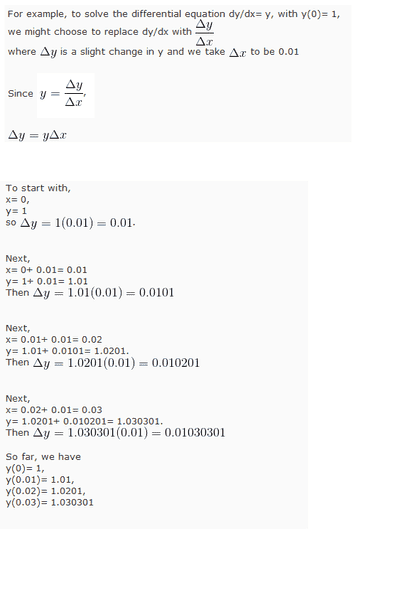is this the same thing we did here ??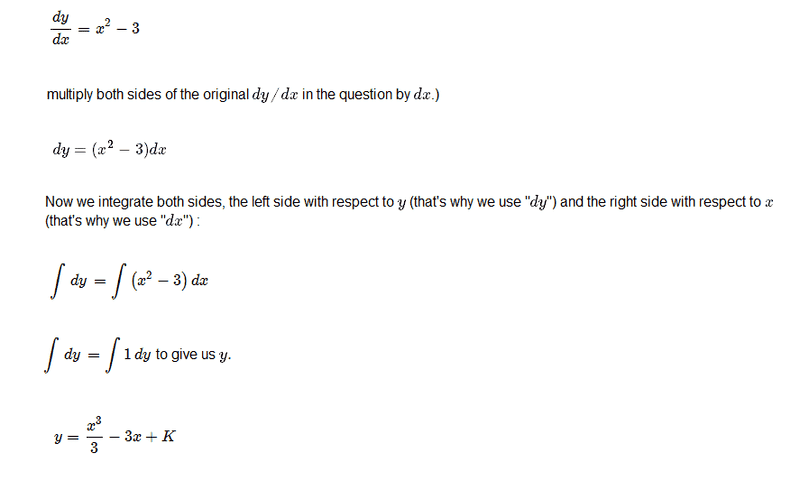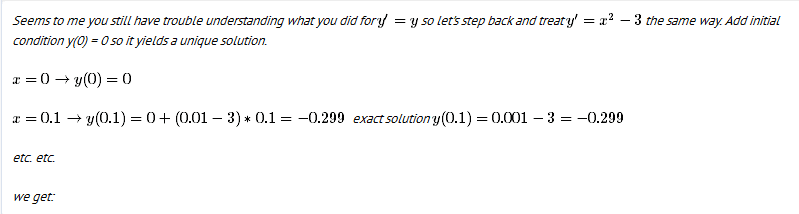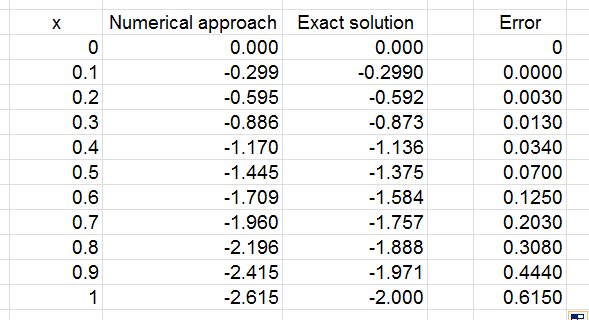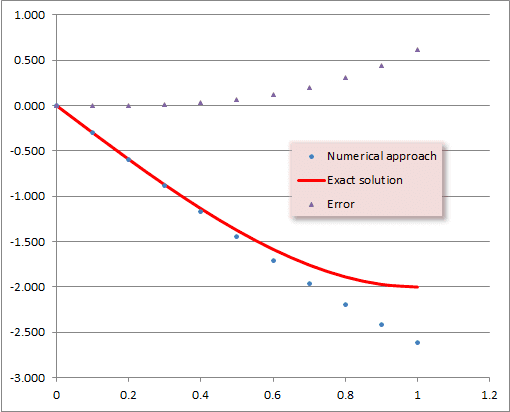first we are given a gradient of the curve or an instantaneous rate of change
then we are trying to find a function that is giving this gradient of the curve or this instantaneous rate of change

then we change the dy/dx , the gradient of the curve or an instantaneous rate of change to ... delta y /delta x
which is an even smaller gradient of the curve or a smaller instantaneous rate of change ...

when we increment x by 0.1 , we get the value of y around -0.299

which is another gradient of the curve for delta y / delta x , the smaller instantaneous rate of change

why are we adding up these gradients of the curve or the smaller instantaneous rate of change for these small small change in delta y / delta x?

this means that these smaller gradients of the curve or these smaller instantaneous rate of change has certain property at each value of x ..., the gradient of the curve with respect to x which is indeed "the rate" at which y is changing as x is changing ....

so the function has the property of this "rate" ???

usually , when we try to solve ...

we get a function that has this instantaneous rate of change ...

but when we try to solve it with numerical methods , we only get a " rate " which is the property of that function ???

BvU
Homework Helper
Again, several feet of post.
how to find a proper initial guess ...?
Good thing you already prepared an answer. General answer: use all your knowledge, intuition and luck. As if you were a TV detective. Stay critical.

second is ...
Numerically, we use the derivative (which changes from point to point) to approximate the function as linear. Which is good up to order (step size)2. After a step, we re-evaluate the derivative. We make errors of a certain order, but still get an estimate of the function values.

Start reading the bookMark44
Mentor
I see two two-foot long posts and I don't see a difference. Nor a question I can answer ...
I deleted the duplicated images

Mark44
Mentor
Start reading the bookThis is advice that has been given in previous threads to you (rosiekidcute). Have you obtained a book, yet?

Last edited:
BvU ,

thanks ...

Mark44 ,

yes , i have this book ... numerical analysis and i am reading it ...

Mark44
Mentor
yes , i have this book ... numerical analysis and i am reading it ...
It would make more sense to start with a textbook that deals with ordinary differential equations.

You have recently started threads with these titles:
• Few basic questions about differential equations...
• A few differential equation questions
• differential equations and numerical methods questions
• first order differential equations ??
• few more numerical methods question...?
Any good differential equations textbook would provide answers for all of the questions you've asked here.

Last edited:
Mark44,

thanks ...

can you recommend some books on ordinary differential equations ??

i have few more doubts from the previous posts ...
i don't know if i am understanding this properly ...
this thread , post # 9 ...
picture two ..

that question is about finding the "function" that has that instantaneous rate of change ... right ??

we found the function ... that has that rate of change ...

picture one ,

we are given a function and we are trying to solve it numerically ...

if you take dy/dx of that instantaneous rate of change
you get certain properties of that function at certain points or places ...
then if you increment x ... you get properties of that function again at certain points or places

if you add all the function values ... you get the overall properties of a function ...
and that is the function with all those properties that has given that instantaneous rate of change ...

??